﻿ Free particle
Quantum Mechanics
Motion in a potential: Free particle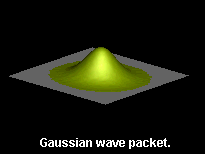In classical mechanics the position and the momentum of a particle are known exactly. In quantum theory, the probability distribution to observe a particle at a certain position has a nonzero width. This implies that repetition of the experiment with the particle (under the same conditions etc.) will yield the average position with a statistical uncertainty, characterized by the variance of the distribution. According to the Heisenberg uncertainty relation, the product of the variance of the position and momentum cannot be made less than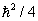. The wave function for which this product is equal tois called the minimum uncertainty wave packet. Such a wave packet takes the form of a Gaussian. In free space (i.e. in the absence of external forces) a Gaussian wave packet moves very much like a classical particle. As time departs from zero in both the past and future directions, the wave packet spreads, but remains Gaussian.

The following animations illustrate the spreading of the wave packet. The initial wave packet is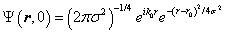where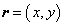,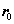denotes the center of the wave packet,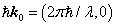is the mean momentum and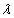sets the length scale.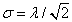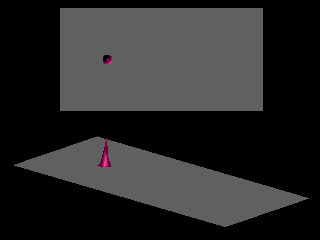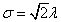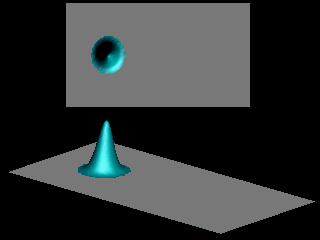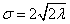The spread of the initial wave packet determines the uncertainty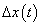on the position of the particle. The more a particle is localized in space at time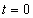the faster its probability distribution spreads out with time, a consequence of the uncertainty principle. The consequences of the uncertainty principle on the spreading of the wave packets is further illustrated by comparing animations for wave packets of different initial width. The next animation shows the superposition of the animations for(in red) and(in transparent blue). For visualization purposes, the maxima of the initial probabilities are chosen to be the same.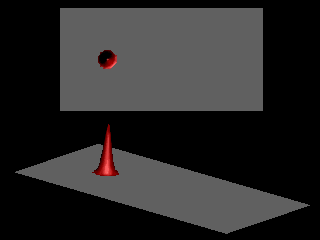Narrow wave packets spread more rapidly than wide packets.

Suppose you could stop time and also reverse the arrow of time. What would happen to the wave packet ? Upon reversing the arrow of time would it

1. continue expanding?
2. contract forever?
3. contract to its initial width and expand again?
4. remain the same?